# 一文学会排列组合

danielmou

0收藏

### 前言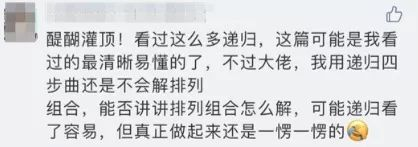1. 什么是排列
2. 排列的常用解法
3. 什么是组合
4. 组合递归解法
5. 面试中排列组合的一些变形

### 什么是排列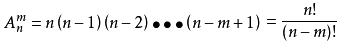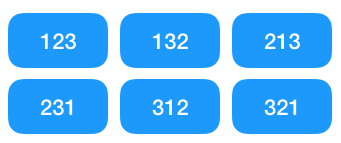### 排列的常用解法

#### 暴力穷举法

``````/**
* 求数字第 1 到 n 的全排列
*/
public     void permutation(    int{
for(    int i = 1; i < n + 1; i ++) {
for(    int j = 1; j < n + 1; j ++) {
for(    int k = 1; k < n + 1; k ++) {
if (i != j && i != k && j != k) {
System.out.println(i + j + k);
}
}
}
}
}``````

#### 递归解题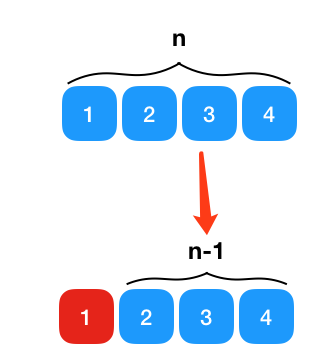1、定义函数的功能 要求数字 1 到 n 的全排列，我们定义以下函数的功能为求从 k 位开始的全排列,数组 arr 存的是参与全排列的 1 到 n 这些数字

``````    public     void permutation(    int{
}``````

2、寻找递推公式 注意上面形成递归的条件：第一个数字已经选中了！那第一位被选中有哪些情况呢,显然有以下几种情况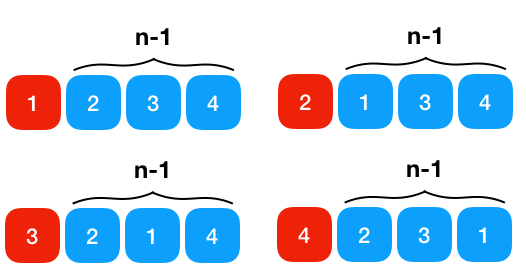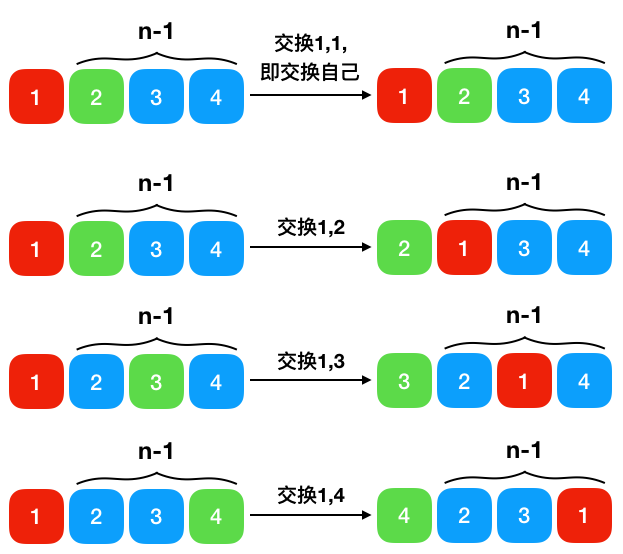3、将第二步的递推公式用代码表示出来补充到步骤 1 定义的函数中，补充后的函数如下

``````/**
*
* @param arr  代表全排列数字组成的数组
* @param k 代表第几位
*/
public     void permutation(    int[] arr,     int{
// 当 k 指向最后一个元素时,递归终止，打印此时的排列排列
if (k == arr.length - 1) {
System.out.println(Arrays.toString(arr));
}     else {
for (    int i = k; i < arr.length; i++) {
// 将 k 与之后的元素 i 依次交换,然后可以认为选中了第 k 位
swap(arr, k, i);
// 第 k 位选择完成后，求剩余元素的全排列
permutation(arr, k+1);
// 这一步很关键：将 k 与 i 换回来，保证是初始的顺序
swap(arr, k, i);
}
}
}
public     static     void swap (    int[] arr,     int i,     int{
int t = arr[i];
arr[i] = arr[j];
arr[j] = t;
}``````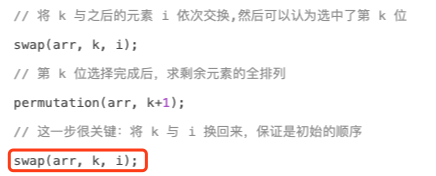4、求时间/空间复杂度 由于我们只用了一个数组 arr，所以空间复杂度显然是 O(n)， 那时间复杂度呢，仔细看上面的编码可以很明显地看出计算 n 的全排列需要做 n 次循环，循环里是要做 2 次交换（由于是固定数字，可以认为是常数 C ），还有一次对之后 n-1 次元素的全排列 所以 f(n) = n * (C + f(n-1))，C是常数可以忽略,所以

f(n) = n * f(n-1) = n * (n-1) * f(n-2) = n!,所以时间复杂度是 O(n!)，注意不可能有比这个更好的时间复杂度了！因为全排列的组合本身就有 n! 次，再怎么优化都肯定会有这么多次

#### 字典序法

1 2 3 ， 1 3 2 ， 2 1 3 ， 2 3 1 ， 3 1 2 ， 3 2 1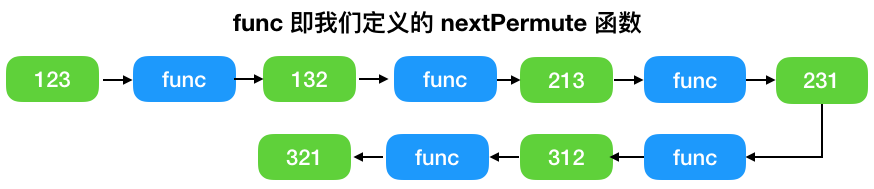1、从右到左（从个位数往高位数）寻找第一个左邻小于右邻的数，如果找不到说明此时的数字为全排列的最大值

2、再从右往左找第一个比第一步找出的数更大的数

3、交换上面两个步骤中的数

4、假设第一步寻找的数对应的位置为 i，则将 i+1至最后一个元素从小到大进行排序，排好序后，此时的数字就是我们要找的那个排列

1、从右到左（从个位数往高位数）寻找第一个左邻小于右邻的数，显然是 4

124653

2、再从右往左找第一个比第一步找出的数（4）更大的数, 显然是 5

124653

3、交换上面两个步骤中的数,即交换 4， 5，此时数字为 125643

4、 125643 中的 643 从小到大进行排序，显然应该为 125346,这一步的排序我们用了快排

``````/**
*
* @param arr   当前排列
* @return boolean 如果还有下一个全排列数，则返回 true, 否则返回 false
*/
public     boolean next_permutation(    int[] arr){
int beforeIndex = 0; //记录从右到左寻找第一个左邻小于右邻的数对应的索引
int currentIndex;
boolean isAllReverse =     true;    // 是否存在从右到左第一个左邻小于右邻的数对应的索引
// 1. 从右到左（从个位数往高位数）寻找第一个左邻小于右邻的数
for(currentIndex = arr.length - 1; currentIndex > 0; --currentIndex){
beforeIndex = currentIndex - 1;
if(arr[beforeIndex] < arr[currentIndex]){
isAllReverse =     false;
break;
}
}
//如果不存在，说明这个数已经是字典排序法里的最大值，此时已经找到所有的全排列了,直接打印即可
if(isAllReverse){
return    false;
}     else {
// 2. 再从右往左找第一个比第一步找出的数更大的数的索引
int firstLargeIndex = 0;
for(firstLargeIndex = arr.length - 1; firstLargeIndex > beforeIndex; --firstLargeIndex) {
if (arr[firstLargeIndex] > arr[beforeIndex]) {
break;
}
}
// 3. 交换 上述 1, 2 两个步骤中得出的两个数
swap(arr, beforeIndex, firstLargeIndex);
// 4. 对 beforeIndex 之后的数进行排序，这里用了快排
quicksort(arr, beforeIndex + 1, arr.length);
return    true;
}
}

public     void swap (    int[] arr,     int i,     int{
int t = arr[i];
arr[i] = arr[j];
arr[j] = t;
}``````

next_permutation 我们写好了，接下来要寻找全排列就容易了，思路如下

1、 首先对参与全排列的数字数组作排序，保证初始的排列数字一定是最小的 即如果起始的 int[] arr = {4,3,2,1} 经过快排后会变成 {1,2,3,4}

2、持续调用定义好的 next_permutation 函数，直到最大值

``````    public     void permutation(    int[] arr){
// 1、 快排，保证 arr 里的元素是从小到大的
quicksort(arr);
// 2、持续调用定义好的 next_permutation 函数，直到最大值
while(next_permutation(arr)) {
System.out.println(Arrays.toString(array));
}
}``````

O(nlog) + O(n! * nlogn) = O(n! * nlogn)。

### 什么是组合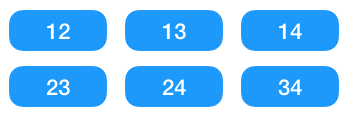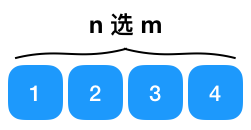• 如果第一个元素选中，则要从之后的 n-1 个元素中选择 m-1 个元素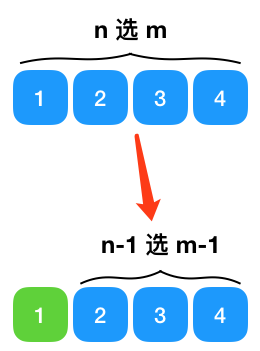• 如果第一个元素未被选中，则需要从之后的 n-1 个元素选择 m 个元素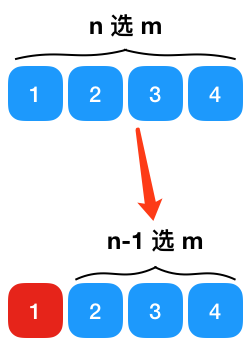1、定义函数的功能 定义以下函数为从数组 arr 中第 k 个位置开始取 m 个元素（如下的 COMBINATION_CNT）

``````    public    static    final    int COMBINATION_CNT = 5;        // 组合中需要被选中的个数
public     static     void combination(    int[] arr,     int k,     int[] select){
}``````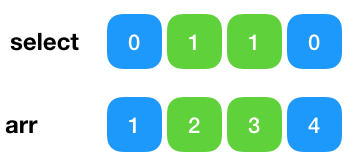2、寻找递推公式 显然递推公式为

``combination(arr, k,m)  = (选中 k 位置的元素 +combination(arr, k+1) ) +  (不选中 k 位置的元素 +combination(arr, k+1) )``

• 一个是被选中的元素已经等于我们要选择的组合个数了
• 一个是 k （开始选取的数组索引） 超出数组范围了。

3、将第二步的递推公式用代码表示出来补充到步骤 1 定义的函数中，补充后的函数如下

``````    public    static    final    int COMBINATION_CNT = 5;        // 组合中需要被选中的个数
public     static     void combination(    int[] arr,     int k,     int[] select){
// 终止条件1：开始选取的数组索引 超出数组范围了
if (k >= arr.length) {
return;
}

int selectNum = selectedNum(select);
// 终止条件2：选中的元素已经等于我们要选择的数组个数了
if (selectNum == COMBINATION_CNT) {
for (    int j = 0; j < select.length; j++) {
if (select[j] == 1) {
System.out.print(arr[j]);
}
}
System.out.print("\n");
}     else {
// 第 k 位被选中
select[k] = 1;
combination(arr, k+1, select);

// 第 k 位未被选中
select[k] = 0;
// 则从第 k+1 位选择 COMBINATION_CNT - selectNum 个元素
combination(arr, k+1, select);
}
}

public     static     void main(String[] args){
int[] arr = {1,2,3,4,5,6,7,8,9};
int[] select = {0,0,0,0,0,0,0,0,0};
// 一开始从 0 开始选 组合数
combination(arr, 0, select);
}``````

4、求时间/空间复杂度 空间复杂度：由于我们用了一个辅助数组 select, 所以空间复杂度是 O(n) 时间复杂度：可以看到 f(n) = 2f(n-1)，所以时间复杂度是O(2^n),显然是指数级别的

### 面试中排列组合的一些变形

1. 在全排列时参与排列的数字都是不相同的， 如果有相同的数字（比如参与排序的是 1， 1，2，3），在使用递归进行解题时，需要进行怎样的改造
2. 在组合中 ，我们的题目是从 n 中选出 m 个数，如果要选出所有组合呢，比如给定 1，2，3，所有的组合是 1, 2, 3, 12, 13, 23, 123, 此时以上的递归解法又该怎么改造帖子
视频
声望
粉丝
社区精华内容